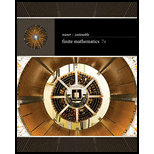# In Exercises 1-42, use Gauss-Jordan row reduction to solve the given systems of equation. We suggest doing some by hand and others using technology. [ HINT: See Examples 1-6.] x + y − z = − 2 x − y − 7 z = 0 0.75 x − 0.5 y + 0.25 z = 14 x + y + z = 4### Finite Mathematics

7th Edition
Stefan Waner + 1 other
Publisher: Cengage Learning
ISBN: 9781337280426

#### Solutions

Chapter
Section### Finite Mathematics

7th Edition
Stefan Waner + 1 other
Publisher: Cengage Learning
ISBN: 9781337280426
Chapter 3.2, Problem 32E
Textbook Problem
1 views

## In Exercises 1-42, use Gauss-Jordan row reduction to solve the given systems of equation. We suggest doing some by hand and others using technology. [HINT: See Examples 1-6.] x + y − z = − 2 x − y − 7 z = 0 0.75 x − 0.5 y + 0.25 z = 14 x + y + z = 4

To determine

To calculate: The solution of the given system of equations,

x+yz=2xy7z=00.75x0.5y+0.25z=14x+y+z=4

By the use of Gauss Jordan row reduction.

### Explanation of Solution

Given Information:

The system of equation is,

x+yz=2xy7z=00.75x0.5y+0.25z=14x+y+z=4

Formula Used:

Elementary row operations

Type 1: Replace the row Ri by aRi, where a is a nonzero number.

Type 2: Replace the row Ri by aRi±bRj, where a is a nonzero number.

Gauss Jordan reduction method:

Step 1: First clear the fractions or decimals if any, using operations of type 1.

Step 2: Select the first nonzero element of the first row as pivot.

Step 3: Use the pivot to clear its column using operations of type 2.

Step 4: Select the first nonzero element in the second row a pivot and clear its column.

Step 5: Select the first nonzero element in the third row a pivot and clear its column.

Step 6: Turn all the selected pivot elements into a 1 using operations of type 1.

Calculation:

Consider the system of equation,

x+yz=2xy7z=00.75x0.5y+0.25z=14x+y+z=4

The augmented matrix for the given system of equations is,

[111210.75110.5170.2510144]

Apply Gauss Jordan reduction method to get the solution of the given system of equation.

Begin by, the simplification of the third row.

Perform the operation R34R3,

[111210.75110.5170.2510144]

Next pivot the first nonzero element of the first row and clear its column.

Perform the operation R2R2R1 and R3R33R1



Next clear the left over element of that column.

Perform the operation R4R4R1.



Next simplify the second and fourth row.

Perform the operation R212R2 and R412R4, 

Next pivot the first nonzero element of the second row and clear its column

### Still sussing out bartleby?

Check out a sample textbook solution.

See a sample solution

#### The Solution to Your Study Problems

Bartleby provides explanations to thousands of textbook problems written by our experts, many with advanced degrees!

Get Started

Find more solutions based on key concepts
f(x)=2xx+1; limxf(x); and limxf(x) x 1 10 100 1000 f(x) x 5 10 100 1000 f(x)

Applied Calculus for the Managerial, Life, and Social Sciences: A Brief Approach

Evaluate each expression: 52+3[ 2(53)+4(4+2)3 ]

Elementary Technical Mathematics

True or False: converges if and only if .

Study Guide for Stewart's Multivariable Calculus, 8th

(f(x)g(x)) = f(x)g(x).

Study Guide for Stewart's Single Variable Calculus: Early Transcendentals, 8th